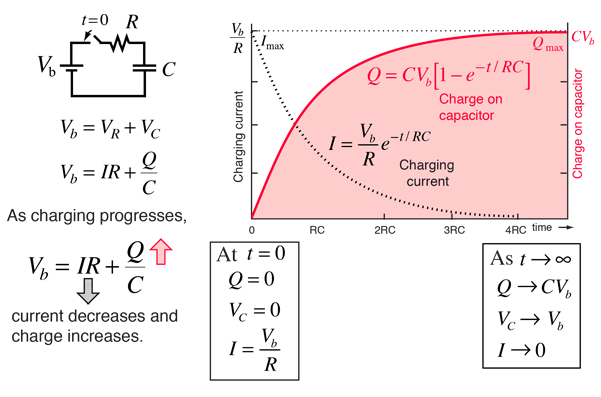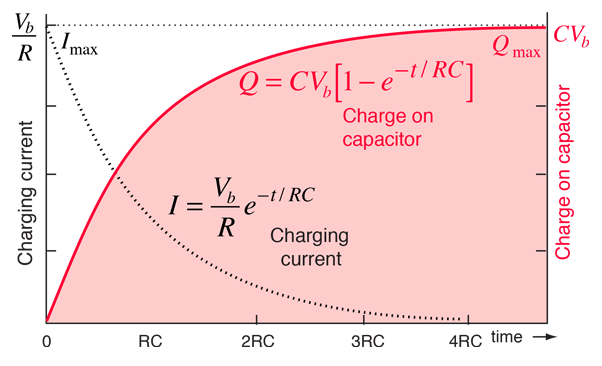# Charging a Capacitor

When a battery is connected to a series resistor and capacitor, the initial current is high as the battery transports charge from one plate of the capacitor to the other. The charging current asymptotically approaches zero as the capacitor becomes charged up to the battery voltage. Charging the capacitor stores energy in the electric field between the capacitor plates. The rate of charging is typically described in terms of a time constant RC.Calculation Derive expressions Capacitor discharge Air tank analogy
Index

DC Circuits

Capacitor Concepts

 HyperPhysics***** Electricity and Magnetism R Nave
Go Back

# Capacitor Charge CalculationFor circuit parameters:
 R = Ω , Vb = V

 C =μF, RC = s = time constant.This circuit will have a maximum current of Imax = A
just after the switch is closed.
 The charge will approach a maximum value Qmax = μC.
 At time t = s = RC
 The charging current is = Imax = A
 and the charge on the capacitor is = Qmax = μC.

 Capacitor charging discussion
Index

DC Circuits

Capacitor Concepts

 HyperPhysics***** Electricity and Magnetism R Nave
Go Back